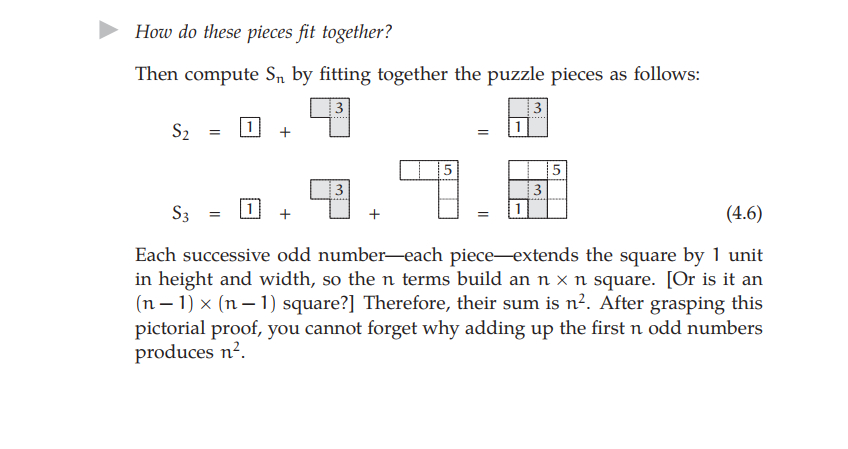# Street-Fighting MathematicsCan you solve a tricky applied mathematics problem in just three seconds? Why is it important to make sure you have units and dimensions right? How can you estimate unknown quantities to a decent approximation? How do you construct simple, yet visually striking, proofs? How do you go from a simple approximation to a better one? Can you solve a very, very difficult problem by solving a simple version of it first, and then applying this solution to the harder problem?

Do you like math but tired of going over complicated proofs and learning specialized techniques that are only useful in a very narrow area of mathematical problems? Would you rather read a mathematics textbook that gives you a powerful toolbox of neat tips and tricks to handle diverse problems in many different areas? That allows you to find good approximate answers to questions that are very difficult to solve exactly? Then this textbook is for you.

Street-Fighting Mathematics is a textbook in mathematical problem solving written by Sanjoy Mahajan and published by MIT Press in 2010. It contains no tedious proofs and no terribly complicated formulas. Instead, it focuses on using math as a useful tool to solve problems using a toolbox of smart tricks. It is available under Creative Commons Attribution–Noncommercial–Share Alike 3.0 United States License.

The book is relatively short (~150) but manages to cover a wide range of issues and teach a variety of problem skills. The first chapter highlights the importance of dimensions when making valid comparisons. It is of vital importance to compare the same dimensions with each other rather than comparing apples and oranges. Perhaps the most vulgar consequence of such failures is the Mars Climate Orbiter that crashed into the planet rather than orbiting it due to confusion between metric and English units. It also goes over the powerful tool of dimensional analysis that allows you to deduce relationship between different variables based on the units working out and being the same on both sides of the equation.

The second chapter applies some of the knowledge learned in the first chapter to a couple of “easy” cases, such as integrals, geometry and fluid dynamics. The third chapter moves on to approximate methods for intelligently estimating various quantities using examples from population demographics, integrals, derivatives, and differential equations. The fourth chapters changes focus to visual proofs of arithmetic and trigonometry, such as bisecting a triangle, geometric means, approximating logarithms, and summations.

The fifth chapter explores successive approximations in areas such as mental multiplication, exponentiation, and even different integrals. Perhaps the most useful example is comparing exact and approximate solutions to the depth of a well. It is a simple problem, but the two different approaches gives different insights.

The sixth and final chapter covers analogy. This is the tool that allows you to make a simpler version of a difficult problem, solve the simpler version and apply the technique you construct in this simpler case to the harder version. The author applies this method in diverse examples, from the bond angle in methane to infinite sums.

The book can be downloaded in PDF format by going here. A cached version of the book can be found here.

#### emilskeptic

Debunker of pseudoscience.